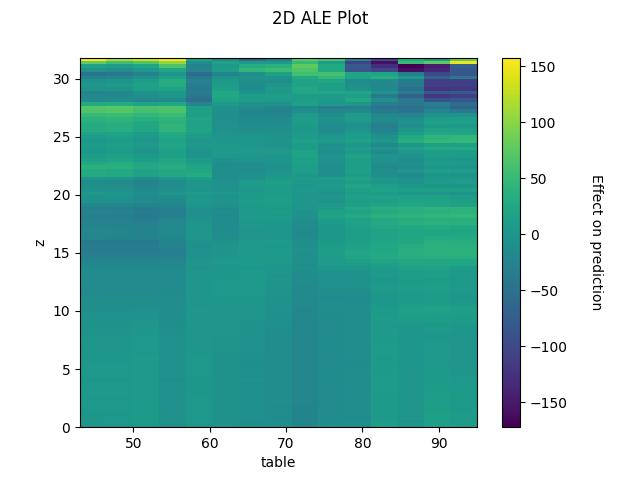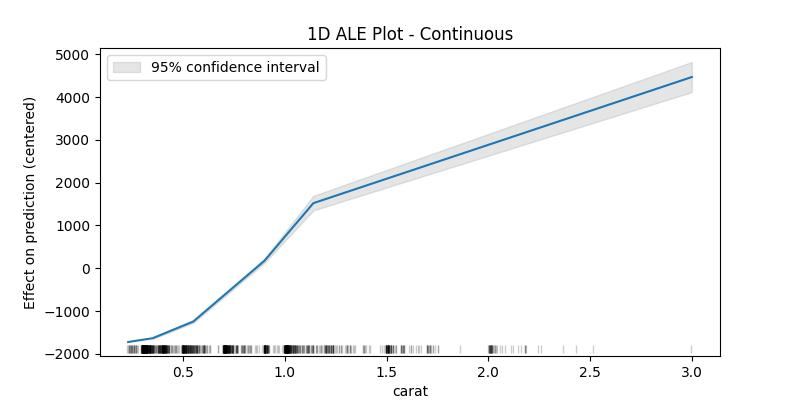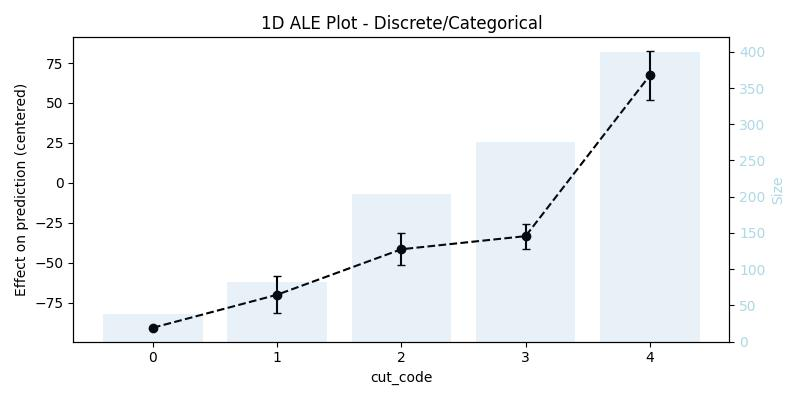# PyALE Release 1.1.2

ALE plots with python

Keywords
ALEPlots, ALE, feature, effect, interpretable, ML
MIT
Install
``` pip install PyALE==1.1.2 ```

# PyALE

ALE: Accumulated Local Effects
A python implementation of the ALE plots based on the implementation of the R package ALEPlot

## Installation:

Via pip `pip install PyALE`

## Features:

The end goal is to be able to create the ALE plots whether was the feature numeric or categorical.

### For numeric features:

The package offers the possibility to

• Compute and plot the effect of one numeric feature (1D ALE)
• including the option to compute a confidence interval of the effect.
• Compute and plot the effect of two numeric features (2D ALE)

### For categorical features:

Since python models work with numeric features only, categorical variables are often encoded by one of two methods, either with integer encoding (when the categories have a natural ordering of some sort e.g., days of the week) or with one-hot-encoding (when the categories do not have ordering e.g., colors). The package offers the option to compute and plot the effect of such features, including the option to compute a confidence interval of the effect. In this case the use has two options:

• For integer encoding: the user can plot the effect of the feature as a discrete feature
• does not need additional preparation steps
• For one-hot-encoding: or any other custom encoding, the package, starting from version 1.1, offers the possibility to pass a custom encoding function to categorical (or string) features.
• in this case the user must provide
• a function that encodes the raw feature
• a data set that includes the raw feature instead of the encoded one (including all other features used for training)
• a list of all predictors used for training the model

The package by default uses the ordering assigned to the given categorical feature, however, if the feature does not have an assigned ordering, then the categories of the feature will be ordered by their similarities based on the distribution of the other features in each category.

## Usage with examples:

• First prepare the data and train a model.

• To explore the different features in this package, we choose one categorical feature to one-hot-encode, and we'll use integer encoding for the rest.
• Full code and other examples can be found in Examples
• For the following examples we train a random forest to predict the price of diamonds with the following data

`X[features]`
carat cut_code clarity_code depth table x y z D E F G H I J
0.23 4 1 61.5 55.0 3.95 3.98 2.43 0.0 1.0 0.0 0.0 0.0 0.0 0.0
0.21 3 2 59.8 61.0 3.89 3.84 2.31 0.0 1.0 0.0 0.0 0.0 0.0 0.0
0.23 1 4 56.9 65.0 4.05 4.07 2.31 0.0 1.0 0.0 0.0 0.0 0.0 0.0
0.29 3 3 62.4 58.0 4.20 4.23 2.63 0.0 0.0 0.0 0.0 0.0 1.0 0.0
0.31 1 1 63.3 58.0 4.34 4.35 2.75 0.0 0.0 0.0 0.0 0.0 0.0 1.0
• import the generic function `ale` from the package

`from PyALE import ale`
• start analysing the effects of your features

Note that the function `ale` has detected the right feature type in all three cases, however, the user can always specify the feature type if she/he thinks that the function did not detect the expected type.

• 2D ALE plot for numeric features

```## 2D - continuous
ale_eff = ale(X=X[features], model=model, feature=["z", "table"], grid_size=100)```## Interpretation:

```random.seed(123)
X_sample = X[features].loc[random.sample(X.index.to_list(), 1000), :]
ale_contin = ale(
X=X_sample,
model=model,
feature=["carat"],
feature_type="continuous",
grid_size=5,
include_CI=True,
C=0.95,
)```For continuous variables the algorithm cuts the feature to bins starting from the minimum value and ending with the maximum value of the feature, then computes the average difference in prediction when the value of the feature moves between the edges of each bin, finally returns the centered cumulative sum of these averages (and the confidence interval of the differences - optional).

`ale_contin`
carat eff size lowerCI_95% upperCI_95%
0.23 -1721.408141 0.0 NaN NaN
0.35 -1633.405685 203.0 -1650.042600 -1616.768770
0.55 -1242.989786 204.0 -1275.489577 -1210.489995
0.90 176.838662 213.0 125.162929 228.514394
1.14 1521.617690 182.0 1351.287932 1691.947448
3.00 4467.185422 198.0 4115.599415 4818.771429

What interests us when interpreting the results is the difference in the effect between the edges of the bins, in this example one can say that the value of the prediction increases by approximately 2946 (`4467 - 1521`) when the carat increases from 1.14 to 3.00, as can be seen in the last two lines. With this in mind we can see that the values of the confidence interval only makes sense starting from the second value (the upper edge of the first bin) and could also be compared with the eff value of the previous row as to give an idea of how much this difference can fluctuate (e.g., for the bin `(1.14, 3.00]` between 2764 and 3127 with 95% certainty).

The lower edge of the first bin is in fact the minimum value of the feature in the given data, and it belongs to the first bin (unlike the following bins which contain the upper edge but not the lower). The size column contains the number of data points in the bin ending with the feature value in the corresponding row and starting with value before it (e.g., the bin `(1.14, 3.00]` has `198` data points). The rug at the bottom of the generated plot shows the distribution of the data points.

For categoricals or variables with discrete values the interpretation is similar and we also get the average difference in prediction, but instead of bins each value will be replaced once with the value before it and once with the value after it.

```ale_discr = ale(
X=X_sample,
model=model,
feature=["cut_code"],
feature_type="discrete",
include_CI=True,
C=0.95,
)```We can also think of it from the perspective of bins as follows, every bin contains two consecutive values (or categories) from the feature, for example with the `cut` feature the bins are `[0, 1]`, `[1, 2]`, `[2, 3]`, `[3, 4]`, and what interests us is still the difference in the effect between the edges of the bins, as well as the range in which this difference fluctuate when taking the confidence interval into consideration. That being said the `size` column contains the number of data points in this category (not the size of the data sample in the bin anymore), this means to get the sample size in the bin one has to sum up the sample size of each value in it (e.g., in `[0, 1]` there is 38 + 82 data points). The bars in the background of the generated plot shows the size of the sample in each category/value.

`ale_discr`
cut eff size lowerCI_95% upperCI_95%
0 -90.799533 38 NaN NaN
1 -70.106933 82 -81.689547 -58.524319
2 -41.629904 204 -51.675662 -31.584146
3 -33.406766 276 -41.209584 -25.603949
4 67.279797 400 52.097705 82.461888

## Development

• Installing the package in edit mode could be done with `pip install -e`
• `unittest` is used for running the tests
• `coverage` is used to get the code coverage, which is not an installation requirement of this package, however will be installed if the dev flag was added to pip call i.e., `pip install -e ".[dev]"`
• To get the code coverage report run `coverage run -m --source=PyALE unittest discover -v` then
• for a fast report in the shell : `coverage report`
• for a detailed html report: `coverage html`
• The versions of the packages used during the development process are freezed in requirements.txt